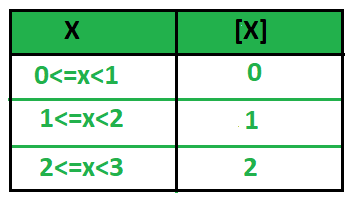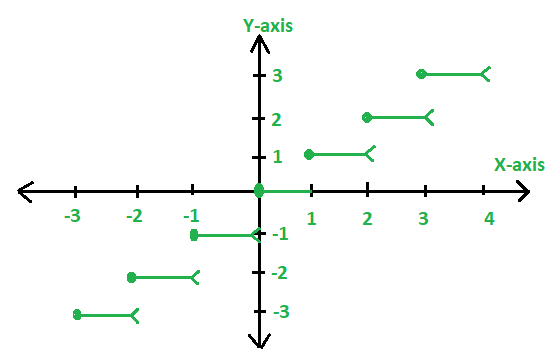# Greatest Integer Function

Greatest Integer Function [X] indicates an integral part of the real numberwhich is nearest and smaller integer to. It is also known as floor of X .

[x]=the largest integer that is less than or equal to x.

In general: If,<=<. Then,Means if X lies in [n, n+1) then the Greatest Integer Function of X will be n.In the above figure, we are taking the floor of the values each time. When the intervals are in the form of [n, n+1), the value of greatest integer function is n, where n is an integer.

1. 0<=x<1 will always lie in the interval [0, 0.9) so here the Greatest Integer Function of X will 0.
2. 1<=x<2 will always lie in the interval [1, 1.9) so here the Greatest Integer Function of X will 1.
3. 2<=x<3 will always lie in the interval [2, 2.9) so here the Greatest Integer Function of X will 2.

Examples:

Input: X = 2.3
Output: [2.3] = 2

Input: X = -8.0725
Output: [-8.0725] = -9

Input: X = 2
Output:  = 2


Number Line Representation

If we examine a number line with the integers and plot 2.7 on it, we see:The largest integer that is less than 2.7 is 2. So [2.7] = 2.

If we examine a number line with the integers and plot -1.3 on it, we see:Since the largest integer that is less than -1.3 is -2, so [-1.3] = 2.

Here, f(x)=[X] could be expressed graphically as:Note: In the above graph, the left endpoint in every step is blocked(dark dot) to show that the point is a member of the graph, and the other right endpoint (open circle) indicates the points that are not the part of the graph.

Properties of Greatest Integer Function:

• [X]=X holds if X is integer.
• [X+I]=[X]+I, if I is an integer then we can I separately in the Greatest Integer Function.
• [X+Y]>=[X]+[Y], means the greatest integer of sum of X and Y is equal sum of GIF of X and GIF of Y.
• If [f(X)]>=I, then f(X) >= I.
• If [f(X)]<=I, then f(X) < I+1.
• [-X]= -[X], If XInteger.
• [-X]=-[X]-1, If X is not an Integer.

It is also known as stepwise function or floor of X.

Below program shows the implementation of Greatest Integer Function using floor():

## C++

 // CPP program to illustrate  // greatest integer Function  #include  using namespace std;     // Function to calculate the  // GIF value of a number  int GIF(float n)  {      // GIF is the floor of a number      return floor(n);  }     // Driver code  int main()  {      int n = 2.3;         cout << GIF(n);         return 0;  }

## Java

 // Java program to illustrate  // greatest integer Function     class GFG{  // Function to calculate the  // GIF value of a number  static int GIF(double n)  {      // GIF is the floor of a number      return (int)Math.floor(n);  }     // Driver code  public static void main(String[] args)  {      double n = 2.3;         System.out.println(GIF(n));  }  }  // This code is contributed by mits

## Python3

 # Python3 program to illustrate   # greatest integer Function   import math     # Function to calculate the   # GIF value of a number   def GIF(n):             # GIF is the floor of a number       return int(math.floor(n));      # Driver code   n = 2.3;      print(GIF(n));          # This code is contributed by mits

## C#

 // C# program to illustrate   // greatest integer Function   using System;     class GFG{   // Function to calculate the   // GIF value of a number   static int GIF(double n)   {       // GIF is the floor of a number       return (int)Math.Floor(n);   }      // Driver code   static void Main()   {       double n = 2.3;          Console.WriteLine(GIF(n));   }   }      // This code is contributed by mits

## PHP

 

Output:

2


Attention reader! Don’t stop learning now. Get hold of all the important DSA concepts with the DSA Self Paced Course at a student-friendly price and become industry ready.

My Personal Notes arrow_drop_upCheck out this Author's contributed articles.

If you like GeeksforGeeks and would like to contribute, you can also write an article using contribute.geeksforgeeks.org or mail your article to contribute@geeksforgeeks.org. See your article appearing on the GeeksforGeeks main page and help other Geeks.

Please Improve this article if you find anything incorrect by clicking on the "Improve Article" button below.

Improved By : Mithun Kumar, ankita_saini

Article Tags :

1

Please write to us at contribute@geeksforgeeks.org to report any issue with the above content.